### MEMO 2017 ekipno problem 6

Kvaliteta:
Avg: 0,0
Težina:
Avg: 6,0

Let$ABC$ be an acute-angled triangle with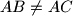$AB \neq AC$, circumcentre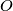$O$ and circumcircle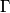$\Gamma$. Let the tangents to$\Gamma$ at$B$ and$C$ meet each other at$D$, and let the line$AO$ intersect$BC$ at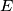$E$. Denote the midpoint of$BC$ by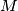$M$ and let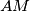$AM$ meet$\Gamma$ again at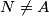$N \neq A$. Finally, let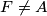$F \neq A$ be a point on$\Gamma$ such that$A, M, E$ and$F$ are concyclic.
Prove that$FN$ bisects the segment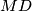$MD$.

Izvor: Srednjoeuropska matematička olimpijada 2017, ekipno natjecanje, problem 6